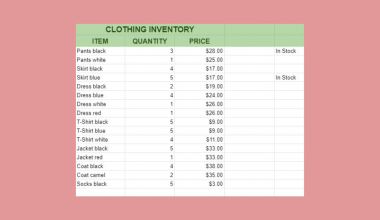How To Use LARGE Function in Google Sheets

The LARGE function in Google Sheets is useful when you need to find out the nth largest element from a data set.

For example, given a dataset of size 100, we can use the LARGE function to find the 10th largest, 50th largest, or 75th largest element in the dataset.

The rules for using the LARGE function in Google Sheets are as follows:

• The function requires two arguments. The first argument is an array or range which holds our dataset. The second argument is the rank of the output to return.
• The function then outputs the nth largest element in the provided dataset.

Let’s look at a scenario where we can use the LARGE function.

Let’s say we have a list of films and their corresponding box office earnings. Using functions like MIN and MAX, we can get the highest-grossing and lowest-grossing films on that list. But what about for cases when we need to know the 2nd, 3rd, or 10th highest-grossing movie? The LARGE function makes it easy to get the nth largest element given a dataset with numerical values.

This use case is just one possible use case for the LARGE function in Google Sheets. The function can be useful to get the nth element of any given range as long as it has numerical data.

Let’s learn how to set up the LARGE function ourselves in Google Sheets. Later, we’ll test out the function with sample numerical values.

The Anatomy of the LARGE Function

The syntax of the LARGE function is as follows:

=LARGE(data, n)

Let’s examine this formula to understand what each of these terms means:

• = the equal sign is how we start any function in Google Sheets.
• LARGE() is our LARGE function. It computes the nth largest element in a dataset.
• data refers to the array or range to consider when using the function.
• n refers to the rank (from largest to smallest) of the element to return.

A Real Example of Using LARGE Function

Let’s look at a real example of the LARGE function being used in a Google Sheets spreadsheet.

In the table below, we have box office data of the top 25 movies from 1999. The films are arranged alphabetically, but we have a breakdown of each film’s worldwide, domestic, and international box office figures.Using the LARGE function, we were able to set up a separate table that pulls up the box office of any arbitrary nth highest-grossing movie worldwide.To fill in the values of worldwide box office, we just need to use the following formula:

=LARGE(\$B\$2:\$B\$26,G3)

The first argument is the range of our worldwide box office column. The second argument is the value of n, which will indicate that the function will return the nth largest number in the range.

If you’re ready to try out the LARGE function in Google Sheets, let’s begin writing it ourselves!

How to Use LARGE Function in Google Sheets

In this section, we will go through each step to start using the LARGE function in Google Sheets. This guide will show you how to use the function to find the fifth highest-grossing movie of 1999 and so on.

Follow these steps to start using the LARGE function:

1. First, select the cell which will hold the result of our LARGE function. In this example, we’ll start with cell H2.2. Next, we just need to input the equal sign ‘=‘ to begin the function, followed by ‘LARGE(‘.
3. You may notice a tooltip box with hints on how to use the LARGE function. Click on the arrow symbol on the top-right-hand corner of the box to minimize it if needed.4. Next, we need to type in our arguments. In the table below, we selected the range that holds the worldwide box office numbers and the cell with the value of n. Afterward, hit Enter on your keyboard to let the function return the nth largest element.5. We can drag down the formula to fill out the rest of the column.6. Additionally, we can use the INDEX and MATCH functions to get the film that corresponds to the returned box office value.1. Can the LARGE function return the nth smallest element instead?
The Large function cannot provide the nth smallest element for a user-defined value n. You may use the SMALL function instead to achieve this task.
2. Why does my formula return a #NUM! error?
The value of n must be between 1 and the size of the dataset. If the value is outside this range, the LARGE formula will return an error.

This function guide shows how easy it is to return the nth largest element in a dataset.

The LARGE function is just one example of a useful function in Google Sheets to work with values in a dataset. With so many other Google Sheets functions out there, you can surely find the functions you need to create the best spreadsheets.

Don’t forget to subscribe to our newsletter to be the first to know about the latest guides and tutorials from us.Our goal this year is to create lots of rich, bite-sized tutorials for Google Sheets users like you. If you liked this one, you'd love what we are working on! Readers receive ✨ early access ✨ to new content.

You May Also LikeHow to Match Multiple Values in a Column in Google Sheets

Knowing how to match multiple values in a column in Google Sheets is useful if you need to…How to Use the IRR Function in Google Sheets

The IRR function in Google Sheets is used to calculate the internal rate of return on an investment…How to Insert Diagonal Line in Cell in Google Sheets

Those who deal with tables in Excel frequently are no stranger to inserting diagonal lines within a cell.…To create a hyperlink to VLOOKUP output cell in Google Sheets is useful if you want to quickly…